•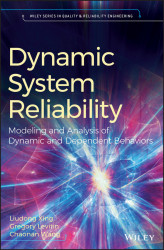#### Dynamic System Reliability#### Overview of this book

This book focuses on hot issues of dynamic system reliability, systematically introducing the reliability modeling and analysis methods for systems with imperfect fault coverage, systems with function dependence, systems subject to deterministic or probabilistic common-cause failures, systems subject to deterministic or probabilistic competing failures, and dynamic standby sparing systems. It presents recent developments of such extensions involving reliability modeling theory, reliability evaluation methods, and features numerous case studies based on real-world examples. The presented dynamic reliability theory can enable a more accurate representation of actual complex system behavior, thus more effectively guiding the reliable design of real-world critical systems. The book begins by describing the evolution from the traditional static reliability theory to the dynamic system reliability theory and provides a detailed investigation of dynamic and dependent behaviors in subsequent chapters. Although written for those with a background in basic probability theory and stochastic processes, the book includes a chapter reviewing the fundamentals that readers need to know in order to understand the contents of other chapters that cover advanced topics in reliability theory and case studies.
PrefaceFree Chapter
Nomenclature1 Introduction2 Fundamental Reliability Theory3 Imperfect Fault Coverage4 Modular Imperfect Coverage5 Functional Dependence6 Deterministic Common‐Cause Failure7 Probabilistic Common‐Cause Failure8 Deterministic Competing Failure9 Probabilistic Competing Failure10 Dynamic Standby SparingIndex# 8.2 PFGE Method

Til and Tip are random variables respectively representing the time‐to‐local‐failure and the time‐to‐PFGE of a system component i. fil(t) and fip(t) represent the probability density function (pdf) of Til and Tip, respectively. qil(t) and qip(t) are unconditional local and propagated failure probabilities of component i at time t, respectively. Thus,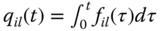qilt=0tfilτdτ--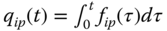qipt=0tfipτdτ--

According to the simple and efficient algorithm (SEA) in Section 3.3.2 [ 2 ,4], the system unreliability can be evaluated based on the total probability law as:

(8.1)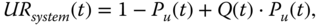UR system t = 1 P u t + Q t · P u t , --

with Pu(t) being defined and computed as

(8.2)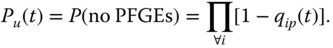P u t = P no PFGEs = i 1 q ip t . --

Q(t) in 8.1 is defined as a conditional system failure probability given that no PFGEs take place during the considered mission time. The evaluation of Q(t) requires...